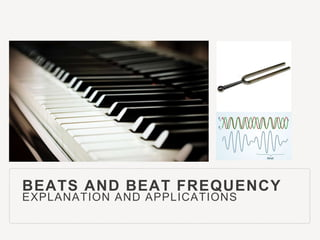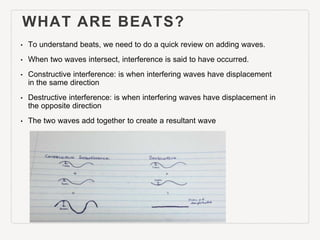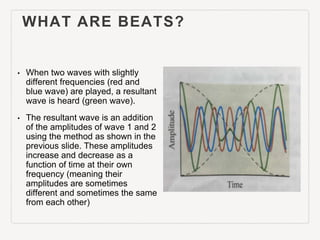Successfully reported this slideshow.×

## Check these out next

This is a Learning object for physics 101 at UBC.This powerpoint presentation explains the concept of beats and beat frequency and includes word problems to further solidify one's understanding.

This is a Learning object for physics 101 at UBC.This powerpoint presentation explains the concept of beats and beat frequency and includes word problems to further solidify one's understanding.

1. 1. BEATS AND BEAT FREQUENCY EXPLANATION AND APPLICATIONS
2. 2. WHAT ARE BEATS? • To understand beats, we need to do a quick review on adding waves. • When two waves intersect, interference is said to have occurred. • Constructive interference: is when interfering waves have displacement in the same direction • Destructive interference: is when interfering waves have displacement in the opposite direction • The two waves add together to create a resultant wave
3. 3. WHAT ARE BEATS? • When two waves with slightly different frequencies (red and blue wave) are played, a resultant wave is heard (green wave). • The resultant wave is an addition of the amplitudes of wave 1 and 2 using the method as shown in the previous slide. These amplitudes increase and decrease as a function of time at their own frequency (meaning their amplitudes are sometimes different and sometimes the same from each other)
4. 4. WHAT ARE BEATS? • Therefore, the resultant amplitude varies. This variation in amplitude is called a BEAT. • As a result of varying amplitude, you hear varying intensities as shown by the dotted black lines.
5. 5. BEAT FREQUENCY • Piano tuners use beats frequency to tune pianos. When the frequencies of a tuning fork and a piano note are getting closer, the beats are slower, until the two sounds converge into one continuous sound when their frequencies are the same. (NOTE: Similarly, when the two sounds have frequencies that are getting further apart, the beats become more rapid until the difference in frequency is so large we hear the sounds as two distinct tones rather than one sound with varying intensities.)
6. 6. LET’S TRY A QUESTION: QUESTION 1 • A tuning fork with a frequency of 450Hz and another tuning fork with a frequency of 453Hz are played simultaneously, how many beats will be heard over a period of 10 seconds?
7. 7. • Hint : The beat frequency is the difference in Hz between the two tuning forks.
8. 8. SOLUTION TO QUESTION 1 • 453Hz- 450Hz = 3Hz. The beat frequency is 3 Hz. • 3Hz means 3 oscillations in a second, therefore in 10 seconds, there will be 3 x 10 = 30 oscillations. In every oscillation you should hear a beat and therefore there will be 30 beats.
9. 9. QUESTION 2 • A tuning fork has a frequency of 440 Hz. Another tuning fork has an unknown frequency. When the two tuning forks are struck simultaneously, they produce a beat frequency of 4Hz. A small piece of play dough is placed on the tuning fork with 440 Hz and its frequency is lowered slightly. Now, when you strike them, they produce a beat frequency of 9Hz. a) What is the frequency of the 440Hz after the play dough is attached to it? b) What is the frequency of the unknown tuning fork?
10. 10. • Hint 1: Remember the beat frequency is the difference in Hz between two wave frequencies.
11. 11. • Hint 1: Hint 1: Remember the beat frequency is the difference in Hz between two wave frequencies. • Hint 2: To calculate part a, can you make two equations and use substitution so the variable of the unknown frequency can disappear?
12. 12. SOLUTIONS TO QUESTION 2 • a)
13. 13. SOLUTIONS TO QUESTION 2 • b)
14. 14. CITED REFERENCE • Physics textbook (for the graph on slides 3 and 4): Physics for Scientists and Engineers - An Interactive Approach
15. 15. THANK YOU FOR WATCHING!